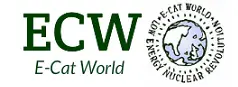# The Power Density of the E-Cat QX

A comment from Alberto on the Journal of Nuclear Physics came up with the following calculation

I understood that the E-Cat reactor has a diameter of 0.08 cm and a length of 0.6 cm. This makes a volume of 0.04^2 x Pi x 0.6 cm^3, which makes 0.003 cubic centimeters. The power that has been measured during the Stockolm test is 20 W. These two numbers make the power density of the E-Cat QX 20 W/0.003 cm^3. This energy density is stunning, it makes a power density of 333 x 20 W/cm^3= 6.6kW/cm^3
Now I understand why you are thinking to a jet engine operated by the E-Cat QX. We are at the eve of a new industrial revolution.

I asked Andrea Rossi if the calculations were correct, and he replied ” the density of power of the E-Cat QX is very high and yes, I think that jet engines or gas engines can be born by this technology. The numbers are what they are.”

If my calculations are correct, converting cm^3 to liters are as follows:

6600kW/l = 6.6MW/l

One point of comparison I have found is from Wikipedia here where it states that Plutonium has a power density of 38,360W/m^3.

In terms of m^3, the E-Cat QX numbers we are working with would be 6.6GW/m^3

Please someone correct me if I am wrong, and maybe there are some people out there who can help to see how this compares to other energy sources.